02/06/2023
by

## Slides on my website## Current status

• ZHAW still only has 1 SKACH project: deep learning for SKA 🔗
• hydrodynamical simulations   ⇿   SKA mock observations
• more projects to come…

## Goal

• compress the knowledge from hydrodynamical and mock simulations to
• map properties from simulations to mock observations
• infer (hidden) astrophysical properties from observables
• explore the usability of various deep learning techniques
for scientific (high-precision) data

## Hydrodynamical simulations

• cosmological & astrophysical processes from first principle
• latest simulations reach (almost) petabyte sizes   ⇾   ideal for deep learning
• dark matter
• gas (HI, HII, H2, He, etc.)
• velocities
• stars
• temperature
• metallicity
• turbulence
• magnetic field strength
• X-ray luminosity
• Credit: IllustrisTNG Collaboration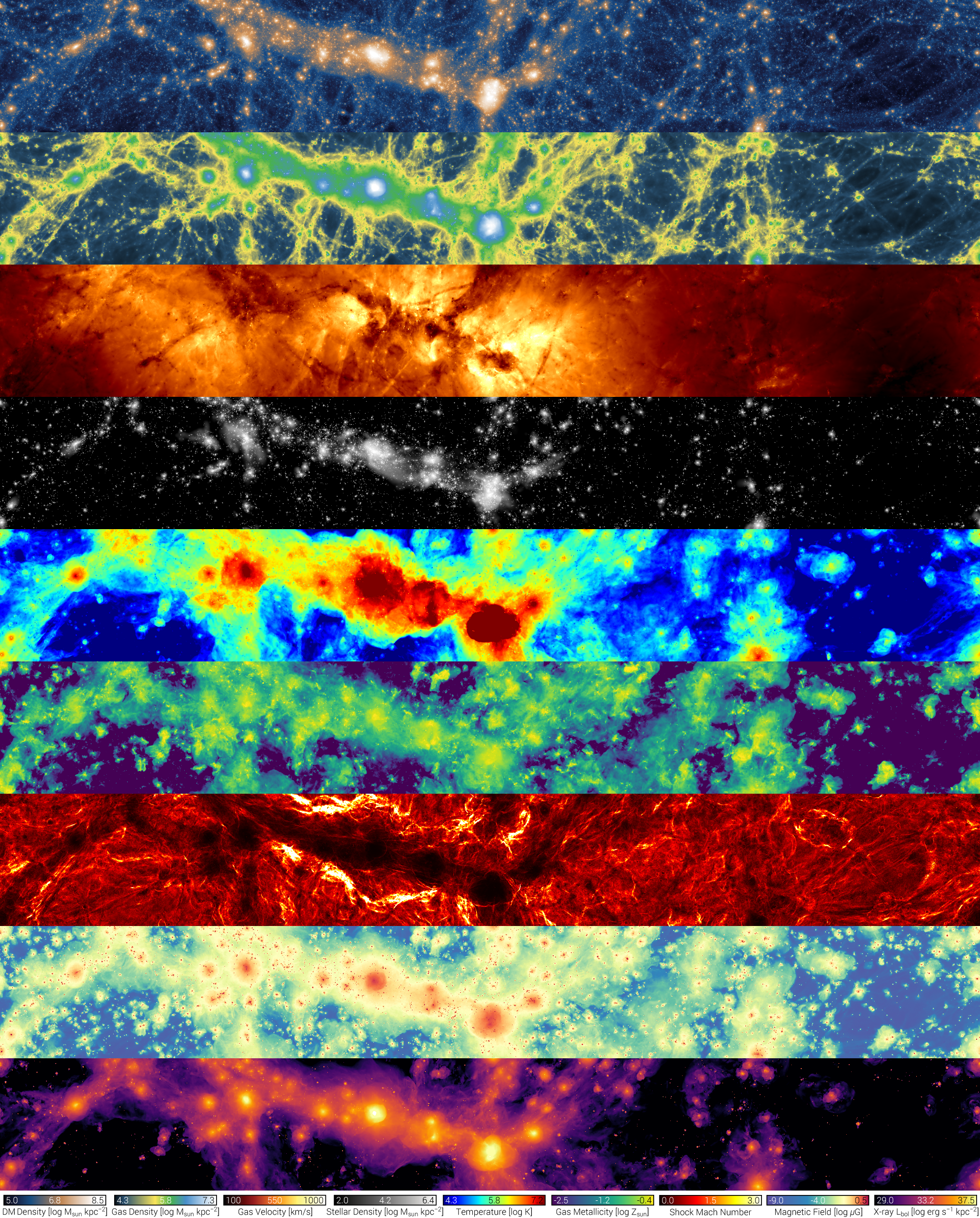## Last time: CycleGAN

• two generator - discriminator pairs
• learn the mapping from domain A   ⇿   B and vice versa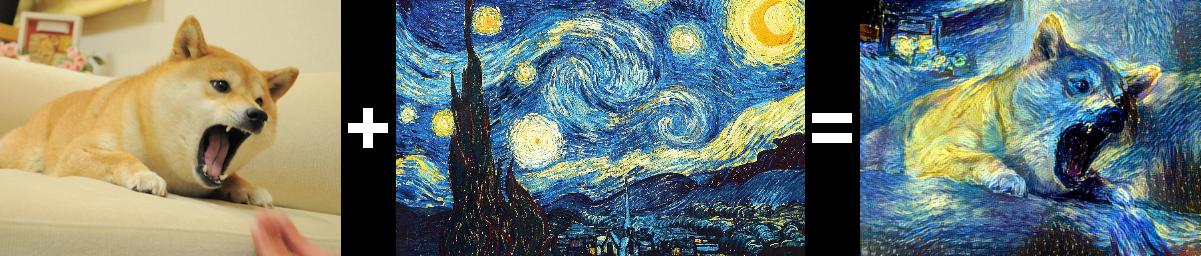## CycleGAN experiments

• dataset: roughly 10'000 galaxies from Illustris TNG50-1
• brightness temperature of the gas   $$T_b(\mathbf{x}) = 189 h \frac{H_0}{a^2H(a)} \frac{\rho_{\text{HI}}(\mathbf{x})}{\rho_c}\,\text{mK}$$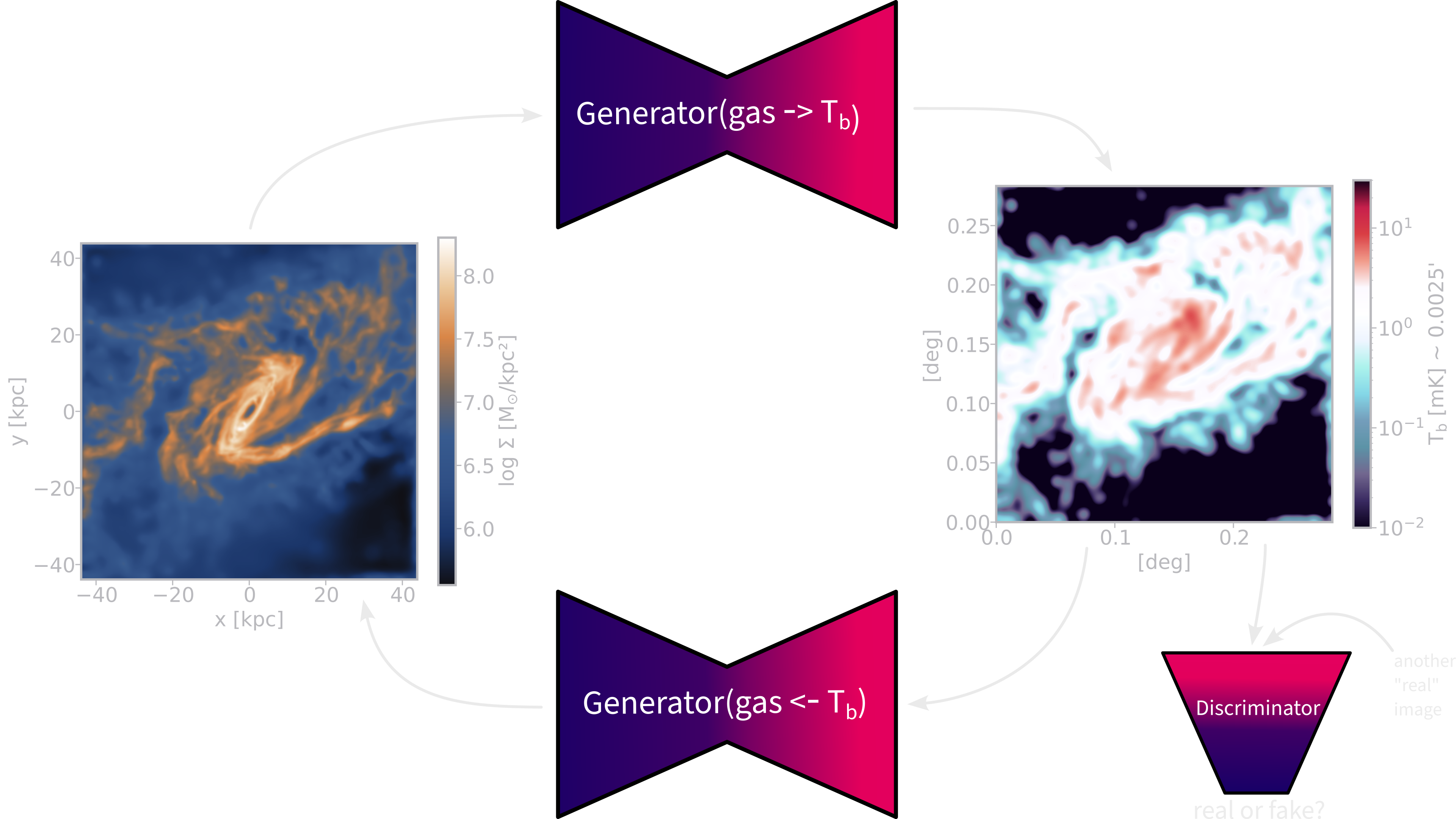## Problem with training GANs

• GANs: $$\quad \mathbb{E}_{x\sim p_\text{data}}[\log{D_\theta(x)}] + \mathbb{E}_{z\sim q(z)}[1-\log{D_\theta(G_\theta(z))}]$$
• fast inference and high quality results
• implicit density and difficult to diagnose
• mode collapse ⇾ not so much an issue for conditional GANs (such as Pix2Pix)

### Failure mode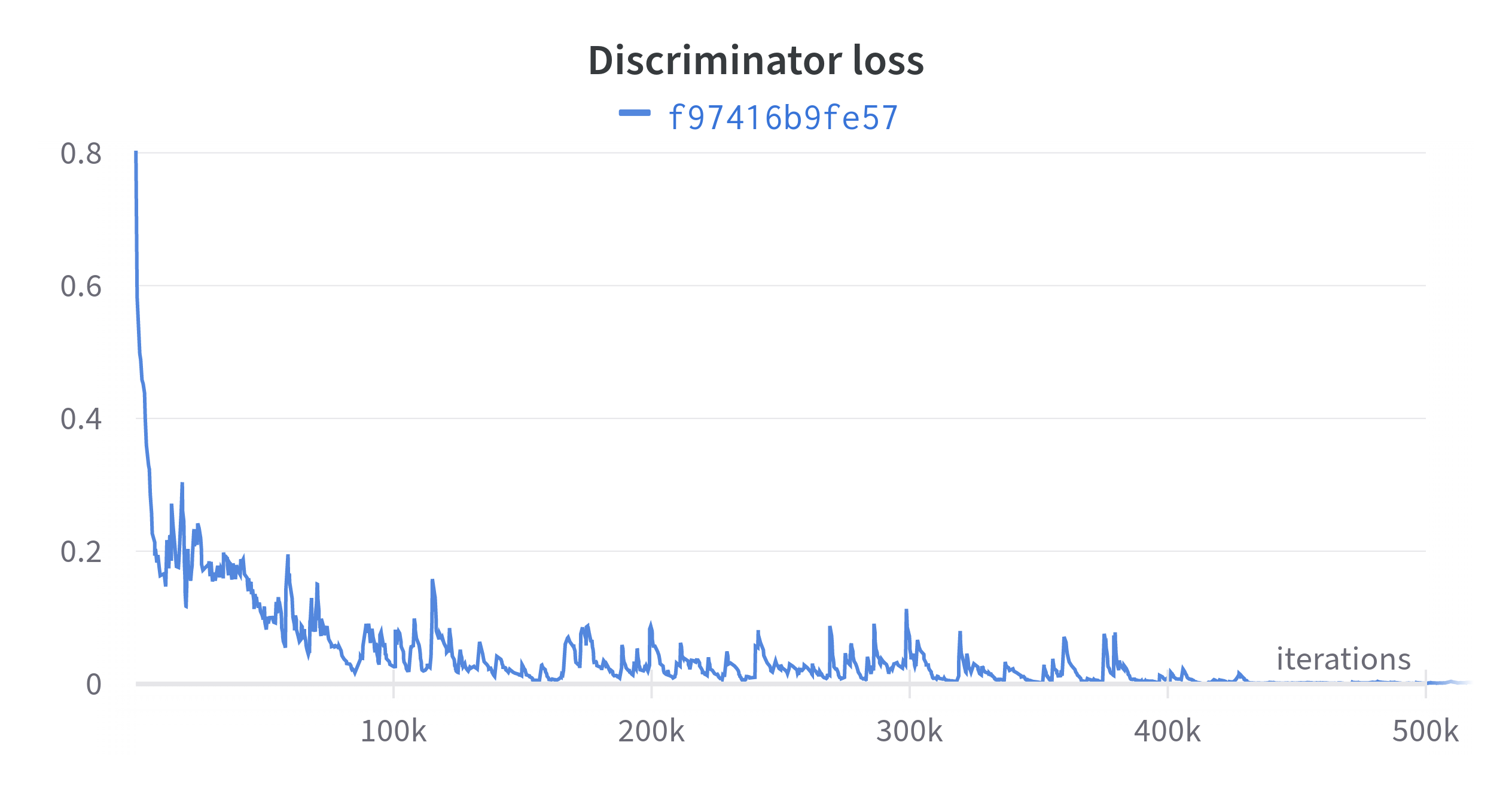Figure 1: Example discriminator loss ending in failure mode

## Pile of data ⇾ AI system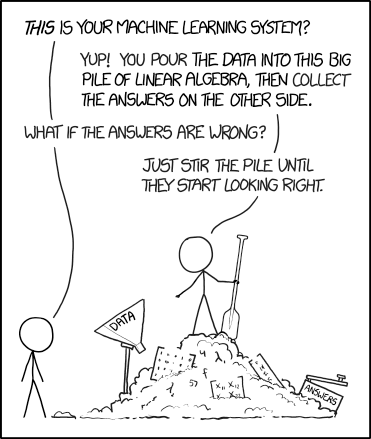Figure 2: https://xkcd.com/1838/

## More parameters, better models?

• hype over generative models: GPT-4, Vicuna, Stable Diffusion, etc.

## Pix2Pix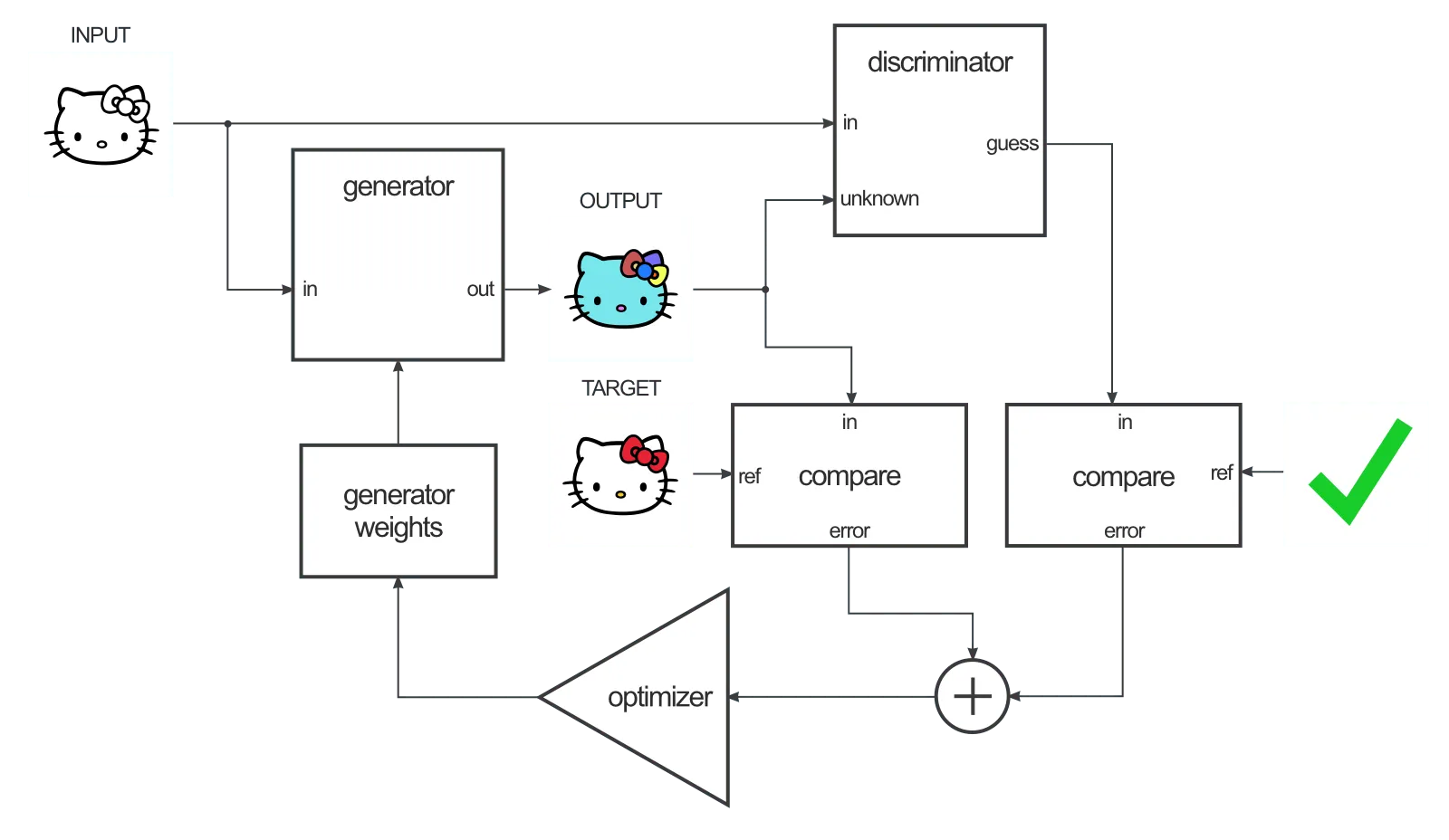Figure 3: Credit: Ch. Hesse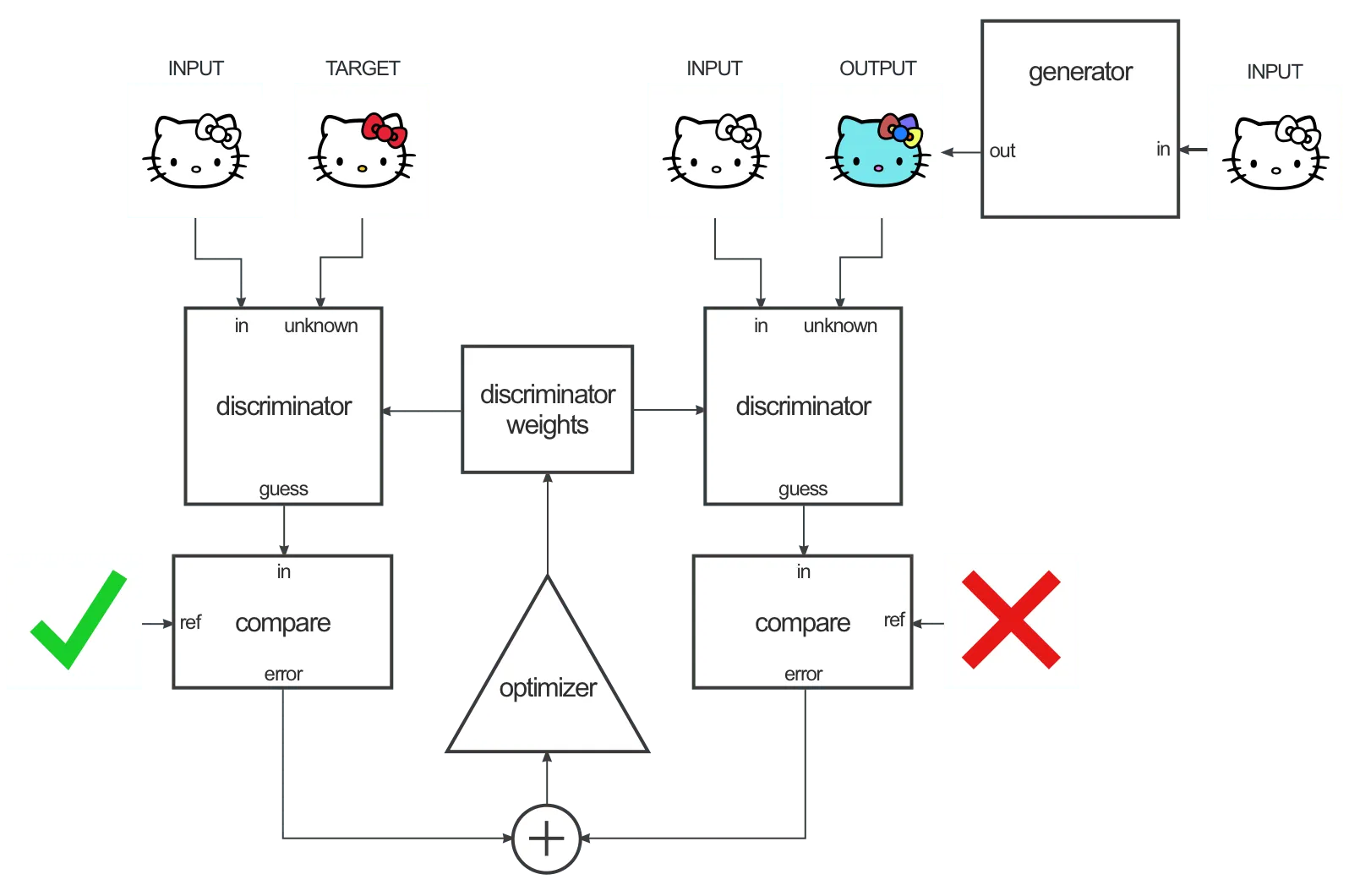Figure 4: Credit: Ch. Hesse

## Domains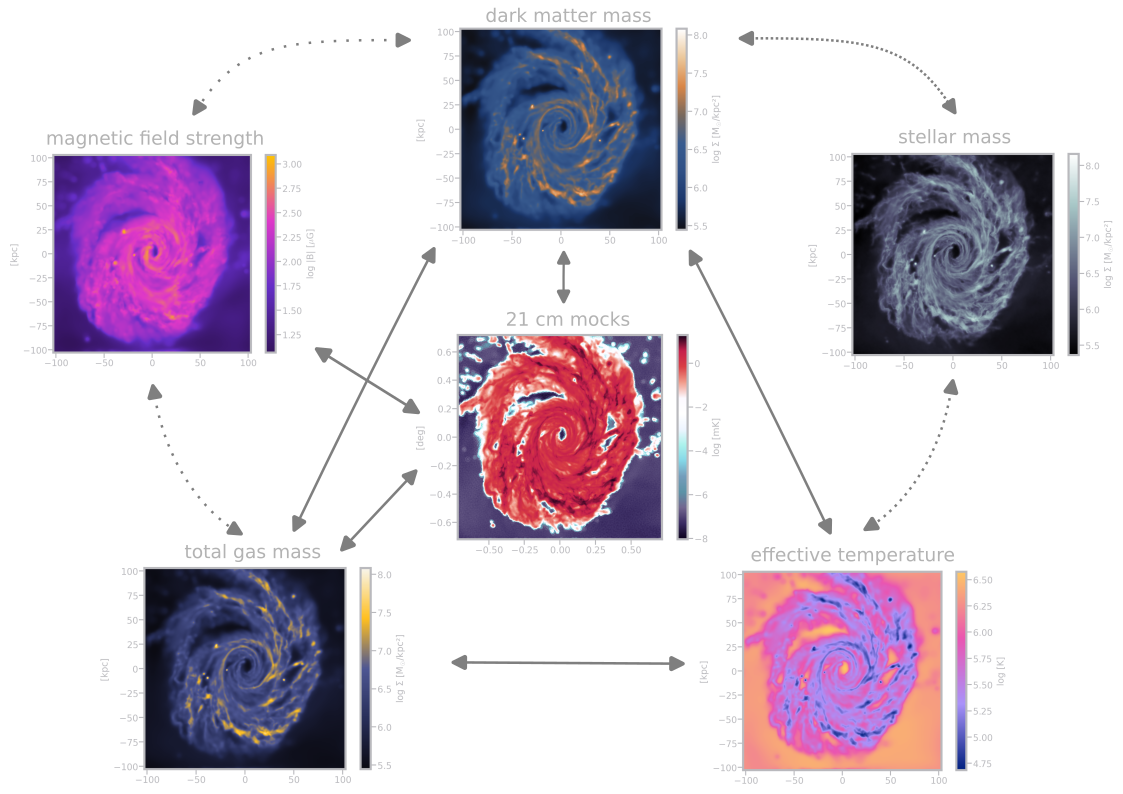Figure 5: current status of our pix2pix network

## Pix2Pix vs. CycleGAN

• tested on a set of 500 TNG50-1 galaxies
• evaluation metric: $$\chi_{\nu}^{2} = \frac{(D_{i,\text{model}} - D_{i,\text{data}})^{2}}{N\sigma_{i}^{2}}$$
(L2 loss normalized with Poisson noise)
domain A domain B CycleGAN Pix2Pix
gas HI 24.47 12.82
HI gas 26.51 13.60
gas 21cm 36.29 (still training)
21cm gas 48.10 (still training)

## Future plans

• better systematics with Karabo
• compare with actual strong gravitational lensing results
• integrate normalizing flow and diffusion networks

Created by phdenzel.